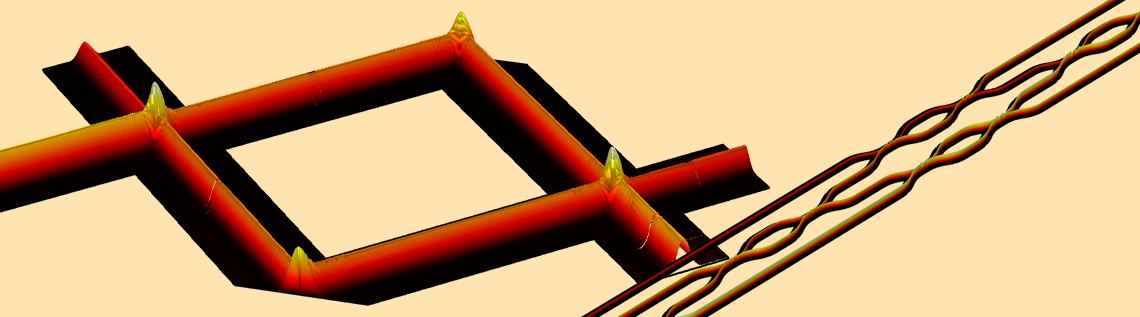# Analyzing an Optical Computation Device with Simulation

August 11, 2022

Optical computing is an alternative paradigm to current electronic computers. In this blog post, we explore the concept of optical computing and explain how an optical matrix multiplication network works. We also discuss how to model an optical computation device using the COMSOL Multiphysics® software and the Wave Optics Module add-on product. Using these products in combination showcases the advantages of applying the beam envelope method when simulating optically large systems.

### Intro to Optical Computing

#### Moore’s Law

There has been exponential growth in the capabilities of computers over the last few decades. This growth obeys Moore’s law, which states that the number of transistors in an integrated circuit will double every two years while the cost of computers will lower. This enables a majority of the modern technologies that we enjoy today. For instance, mainstream computer chips are solely based on electronic components like transistors, and the number of transistors per chip continues to double nearly every two years. To keep up with this increase and to improve the performance of the computer chip at manageable power efficiency, miniaturizing the electronic components on the chip, including the transistors, is both critical and inevitable. Although engineers have been doing a remarkable job in this regard by shrinking the transistor from cm size to nm size, it’s important to realize that, eventually, fundamental limitations will prevent the progression of such devices. For instance, when the size of an electronic component is approaching atomic level, quantum effects will cause instability in its functionality. Scientific and engineering communities have been considering alternative paradigms to electronic computers for a long time. One option that has been drawing a lot of attention recently is optical computing — this is when you perform computation using light (photons) instead of electrical current (electrons).

While optical computing is an emerging technology, optics has been used in information technology for quite some time now, specifically the use of light for information transportation. Optical fibers with extremely low loss can carry information over long distances at the speed of light. Fiber optical network devices are commonly used in data centers and even regular households. However, using light for computation is still in its infancy in terms of commercialization.

#### Mathematical Computation in Optics

It’s well known that certain optical processes correspond to mathematical computations. As an example, let’s consider the diffraction of light. As light passes through a diffractive medium, it’s essentially performing a Fourier transformation integral (a concept we’ll explore in more detail in an upcoming blog post). However, it might not be immediately clear whether an optical system can perform general-purpose mathematical computation like the computers we have today. Currently, optical computing comes in many different flavors. It has been proven that it’s possible to perform simple arithmetics, matrix multiplication, integral and differentiation, and so on using different mechanisms. Generally speaking, analog computation can happen in the diffraction, scattering, or propagation of light in a specifically designed system.

Left: Simulations of the field distribution in a free space Mach–Zehnder interferometer (MZI). Right: An integrated MZI network.

Rather than discussing optical computing in general, we will dive into one particular analog optical computation system: matrix multiplication devices based on the Mach–Zehnder interferometer (MZI) network. This system is very interesting and useful because performing matrix multiplication quickly and in a way that doesn’t require a lot of power consumption is desirable for solving practical problems. This includes problems relating to machine learning. The majority of modern machine learning algorithms, such as deep neural networks, rely on a large amount of matrix multiplication. If we can build an optical system that performs fast matrix multiplication, we can fully harness the power of machine learning.

### Optical Matrix Multiplication

#### Mach–Zehnder Interferometer

First, we need to understand how a single MZI with two inputs and two outputs performs 2-by-2 unitary matrix multiplication. We start with a classical MZI configuration consisting of two 50:50 beam splitters (BS) and three phase shifters, as shown in the figure below. The phase shifts as light passes through the phase shifters by \theta, \alpha, and \beta. We will label the complex amplitudes of the input beams E_1 and E_2. The amplitudes of the output beams are labeled as E’_1 and E’_2.

Next, we will show that E’=U^2(\theta,\alpha,\beta)E where

E= \begin{bmatrix}E_1 \\E_2\end{bmatrix},
E’= \begin{bmatrix}E’_1 \\E’_2\end{bmatrix}

and U^2(\theta, \alpha, \beta) is an arbitrary unitary matrix controlled by \theta, \alpha, and \beta. The superscript, 2 in this case, denotes the dimension of the matrix. We’ll follow this notation convention throughout the post. By controlling \theta, \alpha, and \beta, we can have this optical system performing any unitary 2-by-2 matrix multiplication at the speed of light.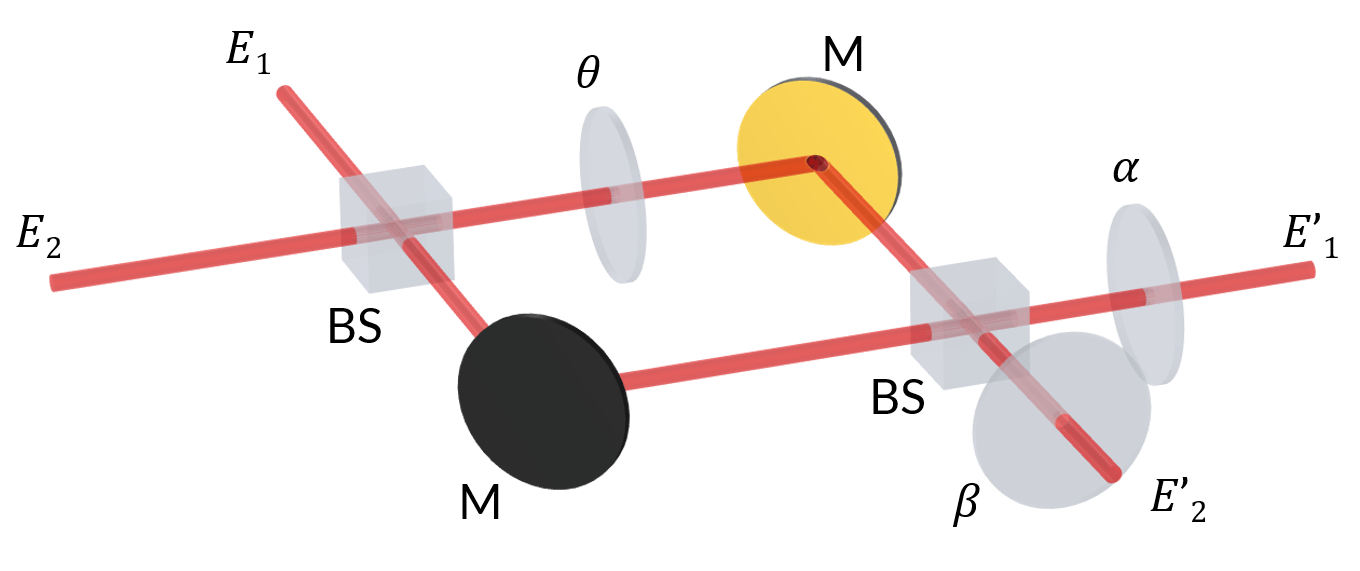A classical MZI with two 50:50 beam splitters and three phase shifters that shift the phase of light by \theta, \alpha, and \beta. M denotes a reflective mirror.

When the beam E_1 goes through a symmetric 50:50 beam splitter, the transmitted beam is \frac{\sqrt{2}}{2}E_1 and the reflected beam is -j\frac{\sqrt{2}}{2}E_1. The appearance of the imaginary number -j in the reflected beam is due to the \pi/2 phase shift on the reflection since e^{-j\pi/2}=-j. For light passing through a beam splitter, say the first one, it picks up a phase factor e^{-j\theta}. Given the information we’ve discussed so far, we can write E’_1 and E’_2 by summing over the light passing through different paths:

E’_1=j\frac{\sqrt{2}}{2}(-j\frac{\sqrt{2}}{2}E_1+\frac{\sqrt{2}}{2}E_2)e^{-j\theta}e^{-j\alpha}-\frac{\sqrt{2}}{2}(\frac{\sqrt{2}}{2}E_1-j\frac{\sqrt{2}}{2}E_2)e^{-j\alpha},
E’_2=-\frac{\sqrt{2}}{2}(-j\frac{\sqrt{2}}{2}E_1+\frac{\sqrt{2}}{2}E_2)e^{-j\theta}e^{-j\beta}+j\frac{\sqrt{2}}{2}(\frac{\sqrt{2}}{2}E_1-j\frac{\sqrt{2}}{2}E_2)e^{-j\beta}

After some algebra, we arrive at

E’_1=\frac{e^{-j\alpha}}{2}[(e^{-j\theta}-1)E_1+j(1+e^{-j\theta})E_2],
E’_2=\frac{e^{-j\beta}}{2}[j(1+e^{-j\theta})E_1+(1-e^{-j\theta})E_2]

In matrix form we have

\begin{bmatrix}E’_1\\E’_2\end{bmatrix}=\frac{1}{2} \begin{bmatrix}e^{-j\alpha}(e^{-j\theta}-1) & je^{-j\alpha}(1+e^{-j\theta})\\je^{-j\beta}(1+e^{-j\theta}) & e^{-j\beta}(1-e^{-j\theta})\end{bmatrix} \begin{bmatrix}E_1\\E_2\end{bmatrix}

We can see that the matrix

U^2(\theta,\alpha,\beta)=\begin{bmatrix}e^{-j\alpha}(e^{-j\theta}-1) & je^{-j\alpha}(1+e^{-j\theta})\\je^{-j\beta}(1+e^{-j\theta}) & e^{-j\beta}(1-e^{-j\theta})\end{bmatrix}

is in the form of the general complex unitary 2-by-2 matrix. It can be easily checked that U^2U^{2*}=I, where I is the identity matrix. Geometrically speaking, this matrix can be interpreted as a rotation for the input vector. Now, how can we model such an optical system in COMSOL Multiphysics?

There are several reasons why we are modeling with the Wave Optics Module. At first glance, the Ray Optics Module might also seem like a good fit since the size of the system is orders of magnitude larger than the wavelength. However, for the MZI, we are predominantly concerned with the interference effect. Ray optics simulation generally doesn’t automatically account for interference, and thus is not an ideal approach.

By using the Wave Optics Module, the interference will automatically be accounted for. We also want to use this module so that we can implement the Electromagnetic Waves, Beam Envelopes interface, which is well suited for handling a model of this size. The beam envelope method is especially suitable for simulating long-distance beam propagation problems as discussed in our previous blog post. By separating the field as the product of a slowly varying envelope function and a rapidly varying phase factor, we only need to mesh the model according to how fast the envelope function varies. This saves us a lot of computational resources in many cases, such as in the MZI shown in the figure above, since the beam is propagating in free space most of the time with no variation in the envelope function. In this system, there are two beam propagation directions — horizontal and vertical. The bidirectional formulation of the beam envelope method is the perfect choice. We set the wave vector with the following settings:

• First wave:
• x = ewbe.k
• y = 0
• Second wave:
• x = 0
• y = -ewbe.k

If we fix \alpha and \beta while gradually varying \theta from 0 to 2\pi, we can investigate the output field amplitudes E’_1 and E’_2. This is done by evaluating ewbe.Ez at the output boundaries. Then we can plot E’_1 and E’_2 in the complex plane, as shown in the figure below. The path traces out a closed loop as \theta changes. This is expected from the derivation we showed earlier. The asterisks in the figure are calculated using the previously mentioned matrix equation, which are consistent with  ewbe.Ez at the output, as expected.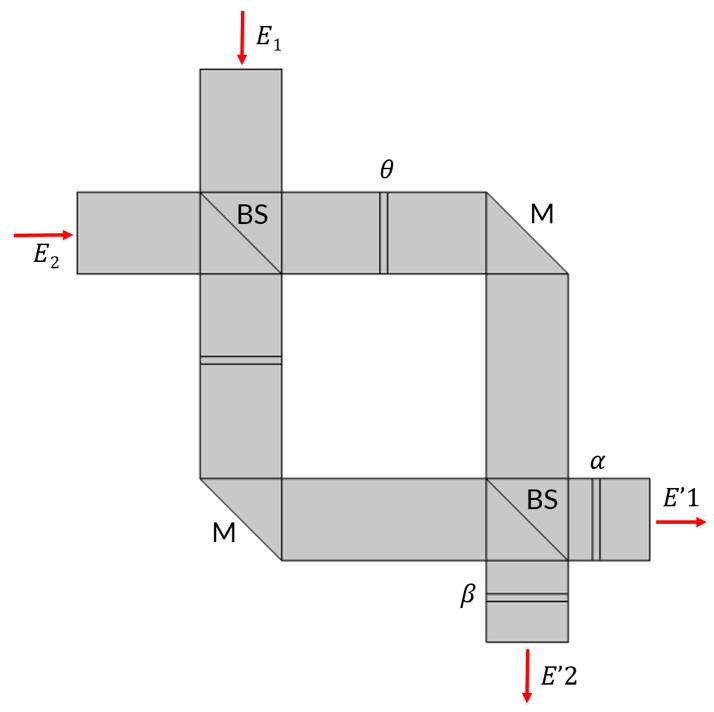Left: A 2D model for a classical MZI with two 50:50 beam splitters (BS) and three phase shifters that shift the phase of light by \theta, \alpha, and \beta. M denotes a reflective mirror. Right: A simulated field distribution as \theta changes from 0 to 2\pi. The input amplitudes are E_1=1V/m and E_2=2V/m.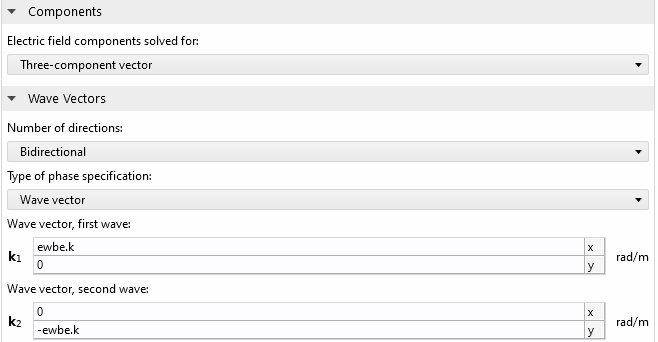The settings for Electromagnetic Waves, Beam Envelopes.

Complex E’_1 (left plot) and E’_2 (right plot) as \theta changes from 0 to 2\pi. The solid curve represents the simulation result and the asterisks denote the expected value calculated using the aforementioned analytical derivation. Horizontal and vertical axes are the real and imaginary parts, respectively, while the colors represent the change in \theta.

#### n-by-n Unitary Matrix Multiplication

It’s fruitful that we now know how to achieve 2-by-2 unitary matrix multiplication, but it’s important to note that in most cases we will be working with matrices much larger in dimension. Now, we will see how we can perform arbitrary n-by-n unitary matrix multiplication using a network of MZIs. Here, we will invoke the theorem that any n-by-n unitary matrix can be written as the product of \frac{n(n-1)}{2} 2-by-2 unitary submatrices. For example, a 4-by-4 unitary matrix U^4 can be written as U^4=R_{31}R_{32}R_{33}R_{21}R_{22}R_{11}, where

R_{11} = \begin{bmatrix} 1 & 0 & 0\\ 0 & 1 & 0\\ 0 & 0 & U^2_1 \end{bmatrix}
R_{22} = \begin{bmatrix} 1 & 0 & 0\\ 0 & U^2_2 & 0\\ 0 & 0 & 1 \end{bmatrix}
R_{21} = \begin{bmatrix} 1 & 0 & 0\\ 0 & 1 & 0\\ 0 & 0 & U^2_3 \end{bmatrix}
R_{33} = \begin{bmatrix} U^2_4 & 0 & 0\\ 0 & 1 & 0\\ 0 & 0 & 1 \end{bmatrix}
R_{32} = \begin{bmatrix} 1 & 0 & 0\\ 0 & U^2_5 & 0\\ 0 & 0 & 1 \end{bmatrix}
R_{31} = \begin{bmatrix} 1 & 0 & 0\\ 0 & 1 & 0\\ 0 & 0 & U^2_6 \end{bmatrix}

Here, U^2_i is a 2-by-2 unitary matrix controlled by one MZI with three phase shifts, as discussed earlier. This matrix decomposition can be intuitively understood by regarding a general rotation in the n-dimensional vector space as a sequence of rotations in lower dimensions. Physically, it means we can build a network of MZIs in the specific sequence where each MZI represents R_{ij}. Thus, the whole system performs arbitrary n-by-n unitary matrix multiplication on the input as light passes through it. In the 4-by-4 unitary matrix case, we need a total of 6 MZIs.

The MZI is equivalent to an optical 2-by-2 unitary matrix multiplication core. It performs 2-by-2 unitary matrix multiplication to the input vector E. The matrix U^2 can be programmed by inducing phase shifts in the MZI via an applied voltage using either the electro-optic effect or thermo-optic effect. In the right plot, the first phase shifter in the MZI is continuously tuned, which induces vector rotation in the outputs.

In principle, this system can be realized using the free space optics like the classical MZI shown in the figure above. However, the scalability of free space optics is rather poor. Beam splitters and mirrors are bulky and nonportable. If we want to build an optical network with a large number of components, a more scalable method is required. Integrated silicon photonics based on current complementary metal–oxide semiconductor (CMOS) fabrication platforms is a promising candidate that is suitable for mass production of highly miniaturized optical components. Analogous to the free space MZI, an integrated MZI based on a waveguide coupler provides the same optical functionality but is 4 orders of magnitude smaller. This allows the possibility of designing optical chips. Designing an MZI with beam 50:50 splitting and sufficient phase shift requires geometry tuning and optimization. We won’t go into detail here, but you can read about how to design a waveguide MZI using the electro-optic effect as the phase shift mechanism in this blog post.

Similarly, the thermo-optic effect is also commonly used to induce modulation of the refractive index and thus the phase shift.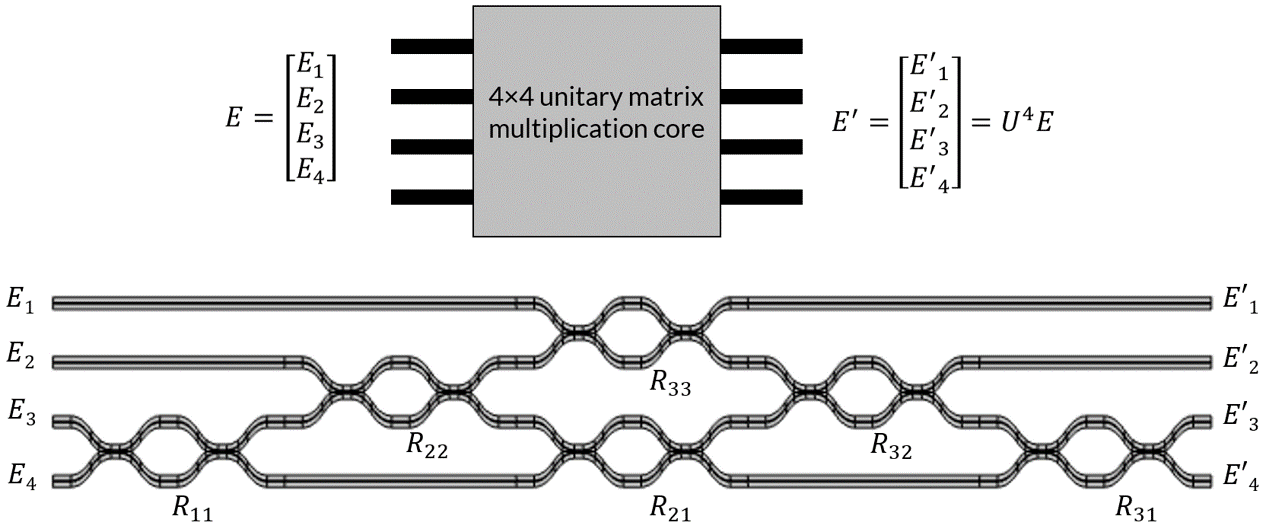An optical 4-by-4 unitary matrix multiplication core. The device consists of a network of 6 MZIs. It performs 4-by-4 unitary matrix multiplication to the input vector. The matrix can be programmed by inducing phase shifts in each MZI using either the electro-optic effect or thermo-optic effect.

The first phase shifter in the first MZI is continuously tuned. This induces vector rotation in the first and second outputs.

#### General n-by-m Matrix Multiplication

So far, we have built up to performing arbitrary n-by-n unitary matrix multiplication using an optical network of \frac{n(n-1)}{2} MZIs. Clearly, n-by-n unitary matrices are a very special class of matrices. To make the system generally useful, we need to tackle the general n-by-m matrix multiplication, which is not limited to only the unitary and square matrix case. This is possible because of the singular value decomposition (SVD). SVD states that any n-by-m matrix M can be factorized as U\Sigma V^*, where U is an n-by-n unitary matrix, \Sigma is an n-by-m diagonal matrix, and V^* is an m-by-m unitary matrix. ^{*} denotes the complex conjugate. Therefore, when computing M, we simply need to have one optical network for U, one optical network for V^*, and connect them with an array of attenuators representing the diagonal matrix \Sigma since diagonal matrix only represents scaling of each element by a constant. The attenuators can also be made of MZIs with a single input and a single output.An optical n-by-m matrix multiplication device consists of two unitary matrix multiplication cores and an attenuator array.

In summary, we have all the ingredients necessary to build an optical system for general n-by-m matrix multiplication. We will link a modeling example for an n-by-n matrix multiplication system below. This model can be used as inspiration for building a more complicated n-by-m matrix.

### Concluding Remarks

In this blog post, we demonstrated that any n-by-m matrix can be factorized as the product of multiple 2-by-2 unitary submatrices and a diagonal matrix. This enables us to build an optical network for general matrix multiplication using a series of MZIs. We have also learned about the advantages of performing optical computation using integrated low-loss silicon photonics.

Will our future cell phones and computers be powered by optical or photonic processors? This awaits to be seen, and there are still many technical difficulties that need to be conquered along the way. What we know for certain is that multiphysics simulation is an essential part in the design and optimization of sophisticated optical computation systems. As seen in this blog post, the beam envelope method feature in COMSOL Multiphysics is particularly suitable for simulating optically large models with fast simulation time and good memory efficiency. It also enables you to simulate the entirety of an optical system, which is crucial when taking other physical effects into account, such as an uneven temperature gradient or mechanical deformation.

### Next Step

Try the Free Space Mach–Zehnder Interferometer and Optical Unitary Matrix Multiplication Device tutorial models yourself by clicking the button below, which will take you to the Application Gallery:

### Further Resources

• Want to read more about Moore’s law, optical computing, and Mach–Zehnder interferometer networks? Check out these related resources:

### References

1. J. Cheng, H. Zhou, and J. Dong, “Photonic Matrix Computing: From Fundamentals to Applications”, Nanomaterials, 11(7), 1683, 2021.

#### Categories##### Hussein Talib
October 4, 2022

Hi,
is there possibility to get the file application in 5.6 version ?##### Tom Chen
October 4, 2022 COMSOL Employee

Hi Hussein,

Unfortunately the models were created in COMSOL Multiphysics 6.0 so we do not have the same models for 5.6. Please consider upgrading your software to 6.0 to view these models.##### Hussein Talib
October 4, 2022

what are the requirements of upgrading ?##### Tom Chen
October 5, 2022 COMSOL Employee

Hi Hussein,##### Hussein Talib
October 5, 2022

ok, so can you provide me with the PDF version of the model like in the other examples?##### Tom Chen
October 5, 2022 COMSOL Employee

Hi Hussein,

Since the detail of the model is described in this article, we don’t have a separate PDF instruction for it. If you have any technical questions regarding this model in particular or using certain software features in general, please contact COMSOL Support and we will be very happy to provide any assistance.##### Hussein Talib
October 5, 2022

ok, I will see.
one last thing, can you provide me with the JAVA file of the model ?##### Tom Chen
October 5, 2022 COMSOL Employee

Hi Hussein,

I think that’s possible. Please file a support case then we can send files in our support system. Thank you.##### Hussein Talib
October 5, 2022

ok, thank you.

EXPLORE COMSOL BLOG